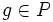# Congruence condition on Sylow numbers in terms of maximal Sylow intersection

This article describes a congruence condition on an enumeration, or a count. It says that in a finite group and modulo prime number, the number of Sylow subgroups, i.e., the number of subgroups of prime power order whose index is relatively prime to the order satisfies a congruence condition.
View other congruence conditions | View divisor relations

## Statement

Let$G$ be a finite group and$p$ a prime dividing the order of$G$. Suppose the intersection of any two distinct$p$-Sylow subgroups has index at least$p^r$ in both of them. Then, if$n_p$ denotes the$p$-Sylow number (?) of$G$, i.e., the number of$p$-Sylow subgroups of$G$, we have:$n_p \equiv 1 \mod p^r$.

In particular,$n_p - 1$ is a multiple of the index of a Maximal Sylow intersection (?).

## Facts used

1. Sylow subgroups exist
2. Fundamental theorem of group actions
3. Intersection of p-subgroup with Sylow subgroup equals intersection with normalizer: If$P$ is a$p$-subgroup of$G$ and$Q$ is a$p$-Sylow subgroup of$G$, then$P \cap Q = P \cap N_G(Q)$.

## Proof

Given: A finite group$G$, a prime$p$ dividing the order of$G$. The intersection of any two distinct$p$-Sylow subgroups has index at least$p^r$ in both of them.

To prove: If$n_p$ is the number of$p$-Sylow subgroups of$G$, then$n_p \equiv 1 \mod p^r$.

Proof: There exists at least one$p$-Sylow subgroup by fact (1). Let$P$ be a$p$-Sylow subgroup. Let$S$ be the set of all$p$-Sylow subgroups.$P$ acts on$S$ by conjugation: in other words, given$g \in P$ and$Q \in S$, we define:$g \cdot Q = gQg^{-1}$.

Suppose$Q \in S$ and$Q \ne P$. Then, the size of the orbit of$Q$ under the action of$P$ is given by (using fact (2)):$\frac{|Q|}{\operatorname{Stab}_P(Q)}$.$\operatorname{Stab}_P(Q)$ is the set of those elements of$P$ that normalize$Q$, and is thus given by$P \cap N_G(Q)$. By fact (3), this intersection equals$P \cap Q$. Hence, its index in$Q$, by assumption is a multiple of$p^r$, and thus, from the above expression, we get that the orbit of$Q$ has size equal to a multiple of$p^r$.

Thus, the orbit of any element in$S$ other than$P$ has size a multiple of$p^r$.$P$ itself, on the other hand, has an orbit of size one. Thus, the total number of members of$S$ is congruent to$1$ modulo$p^r$.